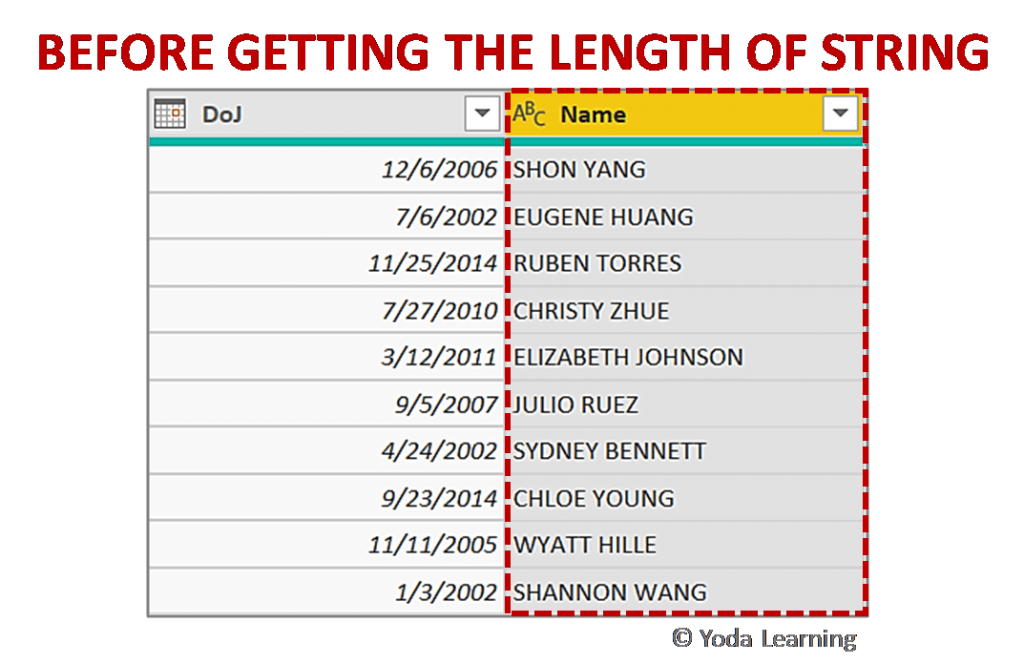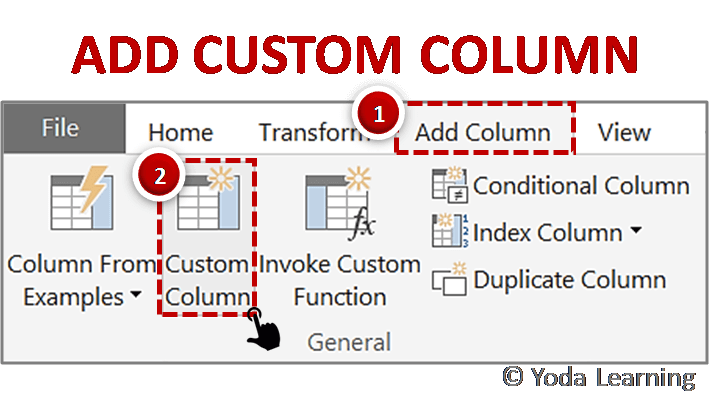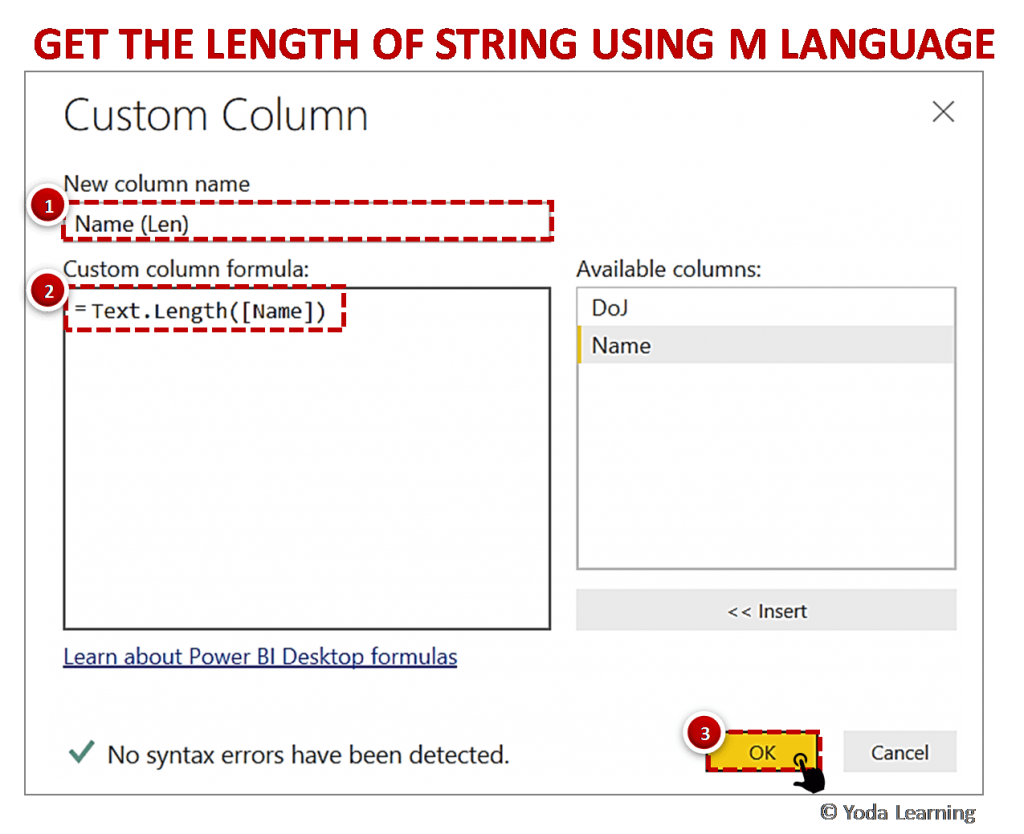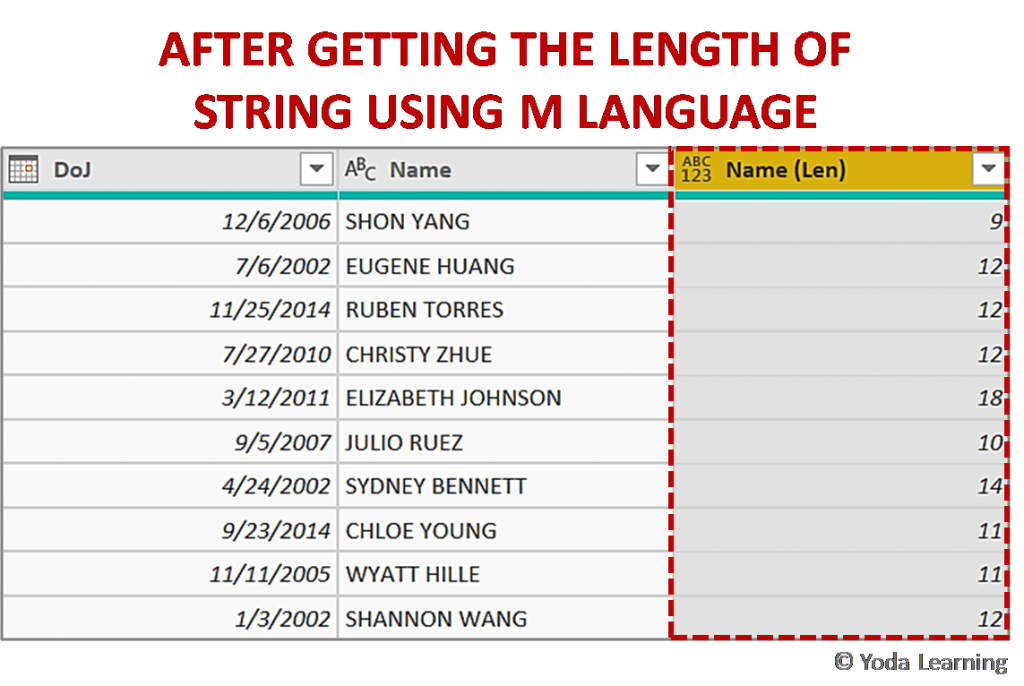# Get The Length Of Strings Using ‘M’ in Power Query

Get the Text Length using Power Query M. If we want to use the Excel LEN function to get the length of strings, it is not supported in M. Let us see how to use the LEN Function in M to get the length of strings.

Data Analytics Tricks in Power BI – Guidebook

You too can analyze data like a Data Scientist. No coding needed. No statistics needed.

Analyze & Visualize data using Power BI. (23 tricks in one book)

## Get Text Length Using ‘M’ Power Query

Follow the below steps to get the Text Length Using ‘M’ Power Query:

### Step 1: Select the Name Column

First, load the data table in Power Query and select the Name column as shown in the below picture. Here, our aim is to get the length of the string from the Name column.### Step 2: Add Custom Column

Add a Custom Column to write a query in M language for getting the length of the string from Name Column. To use a Custom Column feature, go to- Add Column > Custom Column### Step 3: Get the Length of String using M Language

• To get the length of the string we have to create a simple M expression.
• In the New column name text box, we have written Name (Len).
• Now, you can write the M query as given below:
• Text.Length([Name])
• Where,
• Text. Length formula is used to get the length of the Name column
• Click OK to apply.### Step 4: Close & Apply

Close & Apply the Power Query changes after you get the new column added showing the length of string for Name column as given in the below picture.Data Analytics Tricks in Power BI – Guidebook

You too can analyze data like a Data Scientist. No coding needed. No statistics needed.

Analyze & Visualize data using Power BI. (23 tricks in one book)